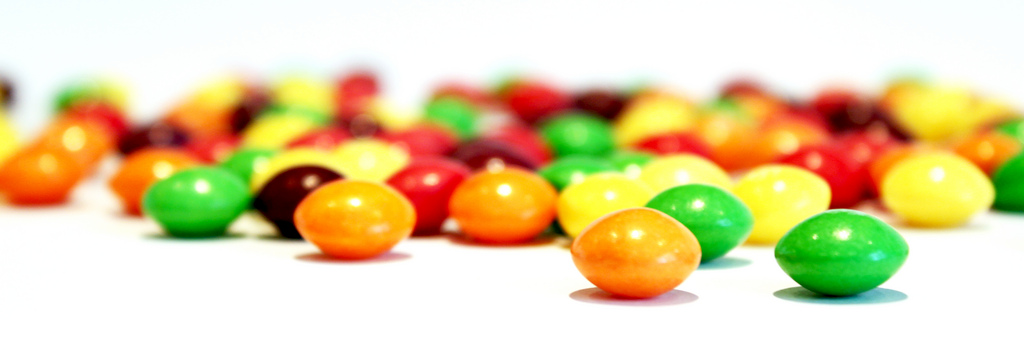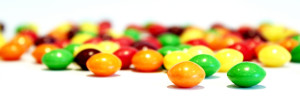n sweets in a bag, some are orange…The other day in the news there was a note about a particular question in one of the national curriculum exams… I thought it was a bit of an odd thing for a maths question to feature in the news and so I thought of having a look a the question. Here it is:

There are$n$ sweets in a bag.

6 of the sweets are orange.

The rest of the sweets are yellow.

Hannah takes at random a sweet form the bag. She eats the sweet.

Hannah then takes at random another sweet from the bag. She eats the sweet.

The probability that Hanna eats two orange sweets is$\frac{1}{3}.$

a) Show that$n^2-n-90=0$

It sounds like an odd question, but after giving it a bit of thought it is actually quite straightforward; and I am glad they ask something that makes you think, rather than something that is purely a mechanical calculation.

So, let’s take a look: Hannah is taking sweets from the bag at random and without replacement (she eats the sweets after all). So we are told that there are 6 orange sweets, so at the beginning of the sweet-eating binge, the probability of picking an orange sweet is:$\displaystyle P(\text{1 orange sweet})=\frac{6}{n}$.

Hannah eats the sweet, remember… so in the second go at the sweets, the probability of an orange sweet is now:$\displaystyle P(\text{2nd orange sweet})=\frac{5}{n-1}$.

Now, they tell us that the probability of eating two orange sweets is$\frac{1}{3}$, so we have that:$\displaystyle \left( \frac{6}{n} \right)\left( \frac{5}{n-1} \right)=\frac{1}{3}$,$\displaystyle \frac{30}{n^2-n}=\frac{1}{3}$,$\displaystyle n^2-n=90$,

which is the expression we were looking for. Furthermore, you can then solve this quadratic equation to find that the total number of sweets in the bag is 10.

The only thing we don’t know is if the sweets are just orange in colour, or also in flavour! We will have to ask Hannah!

This site uses Akismet to reduce spam. Learn how your comment data is processed.